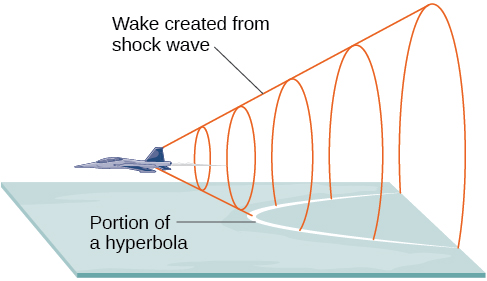12.2 The hyperbola

 Page 1 / 13
In this section, you will:
• Locate a hyperbola’s vertices and foci.
• Write equations of hyperbolas in standard form.
• Graph hyperbolas centered at the origin.
• Graph hyperbolas not centered at the origin.
• Solve applied problems involving hyperbolas.

What do paths of comets, supersonic booms, ancient Grecian pillars, and natural draft cooling towers have in common? They can all be modeled by the same type of conic . For instance, when something moves faster than the speed of sound, a shock wave in the form of a cone is created. A portion of a conic is formed when the wave intersects the ground, resulting in a sonic boom. See [link] .A shock wave intersecting the ground forms a portion of a conic and results in a sonic boom.

Most people are familiar with the sonic boom created by supersonic aircraft, but humans were breaking the sound barrier long before the first supersonic flight. The crack of a whip occurs because the tip is exceeding the speed of sound. The bullets shot from many firearms also break the sound barrier, although the bang of the gun usually supersedes the sound of the sonic boom.

Locating the vertices and foci of a hyperbola

In analytic geometry, a hyperbola    is a conic section formed by intersecting a right circular cone with a plane at an angle such that both halves of the cone are intersected. This intersection produces two separate unbounded curves that are mirror images of each other. See [link] .

Like the ellipse, the hyperbola can also be defined as a set of points in the coordinate plane. A hyperbola is the set of all points $\text{\hspace{0.17em}}\left(x,y\right)\text{\hspace{0.17em}}$ in a plane such that the difference of the distances between $\text{\hspace{0.17em}}\left(x,y\right)\text{\hspace{0.17em}}$ and the foci is a positive constant.

Notice that the definition of a hyperbola is very similar to that of an ellipse. The distinction is that the hyperbola is defined in terms of the difference of two distances, whereas the ellipse is defined in terms of the sum of two distances.

As with the ellipse, every hyperbola has two axes of symmetry . The transverse axis    is a line segment that passes through the center of the hyperbola and has vertices as its endpoints. The foci lie on the line that contains the transverse axis. The conjugate axis    is perpendicular to the transverse axis and has the co-vertices as its endpoints. The center of a hyperbola    is the midpoint of both the transverse and conjugate axes, where they intersect. Every hyperbola also has two asymptotes that pass through its center. As a hyperbola recedes from the center, its branches approach these asymptotes. The central rectangle of the hyperbola is centered at the origin with sides that pass through each vertex and co-vertex; it is a useful tool for graphing the hyperbola and its asymptotes. To sketch the asymptotes of the hyperbola, simply sketch and extend the diagonals of the central rectangle. See [link] .

In this section, we will limit our discussion to hyperbolas that are positioned vertically or horizontally in the coordinate plane; the axes will either lie on or be parallel to the x - and y -axes. We will consider two cases: those that are centered at the origin, and those that are centered at a point other than the origin.

The sequence is {1,-1,1-1.....} has
how can we solve this problem
Sin(A+B) = sinBcosA+cosBsinA
Prove it
Eseka
Eseka
hi
Joel
June needs 45 gallons of punch. 2 different coolers. Bigger cooler is 5 times as large as smaller cooler. How many gallons in each cooler?
7.5 and 37.5
Nando
find the sum of 28th term of the AP 3+10+17+---------
I think you should say "28 terms" instead of "28th term"
Vedant
the 28th term is 175
Nando
192
Kenneth
if sequence sn is a such that sn>0 for all n and lim sn=0than prove that lim (s1 s2............ sn) ke hole power n =n
write down the polynomial function with root 1/3,2,-3 with solution
if A and B are subspaces of V prove that (A+B)/B=A/(A-B)
write down the value of each of the following in surd form a)cos(-65°) b)sin(-180°)c)tan(225°)d)tan(135°)
Prove that (sinA/1-cosA - 1-cosA/sinA) (cosA/1-sinA - 1-sinA/cosA) = 4
what is the answer to dividing negative index
In a triangle ABC prove that. (b+c)cosA+(c+a)cosB+(a+b)cisC=a+b+c.
give me the waec 2019 questions
the polar co-ordinate of the point (-1, -1)ByBy Lakeima RobertsBy Jordon Humphreys